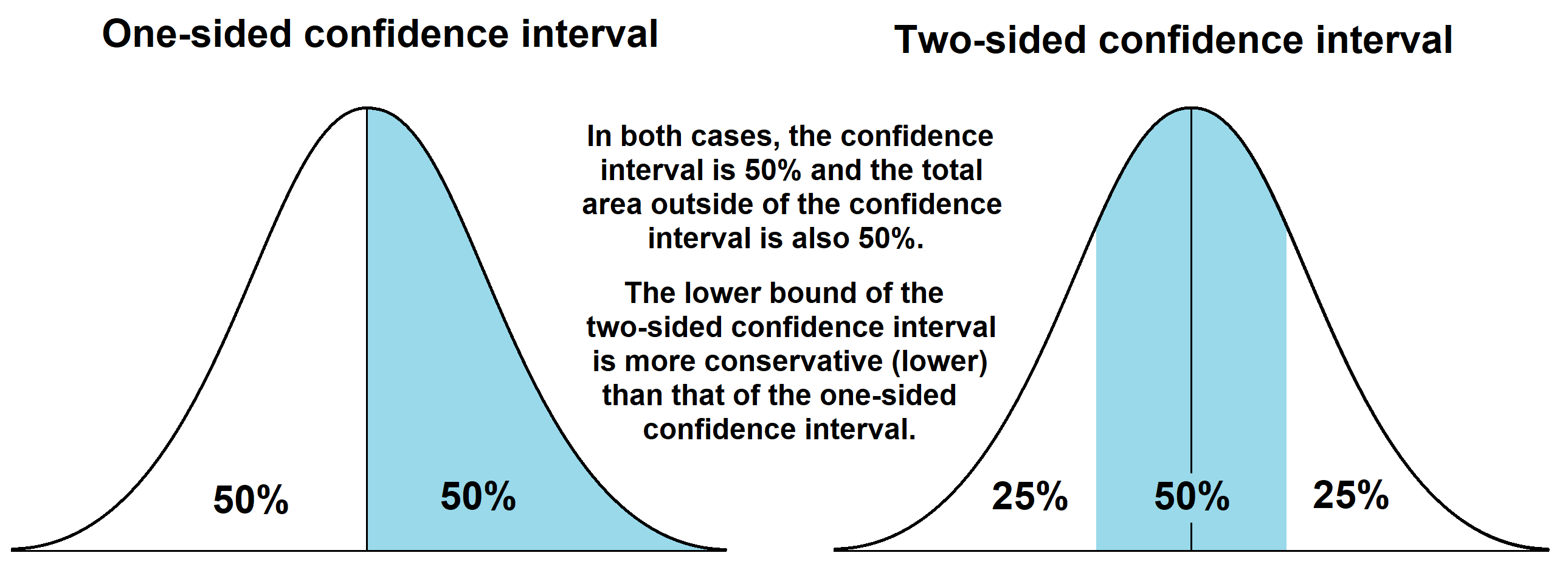# Reliability test planner¶

This function provides a solver to determine the parameters of a reliability test when given 3 out of the 4 unknowns (lower confidence bound on MTBF, test duration, number of failures, confidence interval).

The underlying assumption is that the failures follow an exponential distribution (ie. failures occur randomly and the hazard rate does not change with age). Using this assumption, the Chi-squared distribution is used to find the lower confidence bound on MTBF for a given test duration, number of failures, and specified confidence interval using the formula:

$$MTBF = \frac{2T}{\chi^{2}\left(\frac{(1-CI)}{n},2F+p\right)}$$

Where:

• MTBF = Mean time between failures (same as mean time to failure (MTTF) when the hazard rate is constant as it is here). Note that this is the lower confidence interval on MTBF. If you want the point estimate then specify CI=0.5 and one_sided=False.
• T = Test duration (this is the total time on test across all units being tested)
• CI = Confidence interval (the confidence interval to be used for the lower bound on the MTBF)
• F = number of failures during the test
• n = adjustment for one sided (n=1) or two sided (n=2) test
• p = adjustment for time terminated (p=2) or failure terminated (p=0) test

The above formula can be rearranged, or solved iteratively to determine any of these 4 parameters when given the other 3. The user must specify any 3 out of the 4 variables (not including one_sided, print_results, or time_terminated) and the remaining variable will be calculated. Note the difference between the one-sided and two-sided confidence intervals which are specified using the input one_sided=True/False described below. A description of the difference between one-sided and two-sided confidence intervals is provided at the end of this page. The formula above can be used for either a time terminated test (where the test was stopped at a particular time which was not related to the number of failures) or a failure terminated test (where the test was stopped after a particular number of failures such as all items failing). This setting is controlled using the argument time_terminated which defaults to True.

A similar calculator is available in the reliability analytics toolkit.

API Reference

For inputs and outputs see the API reference.

## Example 1¶

In the example below, we have done a time-terminated reliability test for 19520 hours (units are not important here as it may be days, cycles, rounds, etc.). During the test there were 7 failures. We want to know the MTBF that was achieved during the test within an 80% confidence (two-sided).

from reliability.Reliability_testing import reliability_test_planner
reliability_test_planner(test_duration=19520,CI=0.8,number_of_failures=7, one_sided=False)

'''
Reliability Test Planner results for time-terminated test:
Solving for MTBF
Test duration: 19520
MTBF (lower confidence bound): 1658.3248534993454
Number of failures: 7
Confidence interval (2 sided): 0.8
'''


## Example 2¶

In this second example we want to know the lower confidence bound on the MTBF for a reliability test that has just been conducted. During this test there were 6 failures observed over the 10000km test track and our desired confidence interval is 80%. Note that the output is supressed by setting print_results=False and then the MTBF is accessed by name from the output object.

from reliability.Reliability_testing import reliability_test_planner
output = reliability_test_planner(number_of_failures=6, test_duration=10000, CI=0.8, print_results=False)
print(output.MTBF)

'''
1101.8815940201118
'''


## One-sided vs two-sided confidence interval¶

The below image illustrates the difference between one-sided and two-sided confidence interval. You can use either the one-sided or two-sided interval when you are seeking only the lower bound, but it is essential to understand that they will give very different results for the same CI. They will give equivalent results if the CI is set appropriately (eg. 90% one-sided is the same as 80% two-sided). If you are unsure which to use, the more conservative approach is to use the two-sided interval. If you want the point estimate, use the one-sided interval with a CI=0.5.